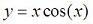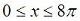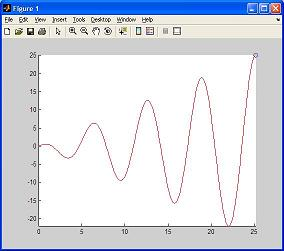# Comet Plotcomet(a) displays an animated comet plot of the vector a.
comet(a, b) displays an animated comet plot of vector b vs. a.
comet(a, b, p) uses a comet of length p*length(b).  Default is p = 0.10.

Example:

If you want to plot the following function:, for the range,  you can write this script in Matlab:

% Clears variables, command window, and closes all figures
clc; clear; close all

% Generates 300 linearly spaced points from 0 to 8*pi
x = linspace(0, 8*pi, 300);

% Creates the formula to be plotted
% (it's a multiplication between vector 'x' and vector 'cos(x)')
y = x .* cos(x);

% Plot it!
comet(x, y, .6)

An the result is...It is much better to see it on your own screen, because it moves like a ribbon!

You can experiment with different p values to see the length of the comet changing...

From 'Comet Plot' to home

From 'Comet Plot' to 'Matlab Plots'

 Top Discrete plots: stems Another way of 2D simple animation 3D animation